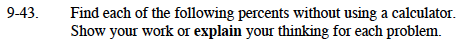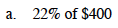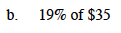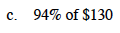### Home > MC1 > Chapter 9 > Lesson 9.1.4 > Problem9-43

9-43.Think of this as 2(10% of $400) + 2(1% of$400).

10% of $400 is 40. 1% of$400 is 4.Think of this as 2(10% of $35) − (1% of$35).

10% of $35 is 3.5. 1% of$35 is 0.35.Think of this as 9(10% of $130) + 4(1% of$130).

What is 10% of $130? What is 1% of$130?